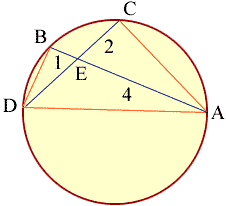Quandaries and Queries Name: Lori Grade level: Geometry(High school) Asked by: OAT quantitative reasoning section Question: Chords AB and CD of circle O intersect at E. If AE=4, AB=5, CE=2, Find ED. Hi Lori, I drew a diagram of the situation you described.I joined AD to form a chord and then drew the line segments AC and BD. Angles ABD and ACD are subtended at the circumference by the same chord so the angles are equal. Also angles CEA abd DEB arre equal so triangles ACE and DEB are similar. Can you now complete the problem? Penny Go to Math Central